Physic Tutorials

# Notes on Dynamics for CIE A level Physics

This is a short note on dynamics which includes motion and conservation of linear momentum for CIE A level Physics.

4.1 Momentum and Newton’s laws of motion

a) understand that mass is the property of a body that resists change in motion

Mass: is a measure of the amount of matter in a body, and is the property of a body which resists change in motion

In kinematics, the motion of a body is independent of its mass, it is a change in its state of motion that is affected by/ depends on its mass

b) recall the relationship F = ma and solve problems using it, appreciating that acceleration and resultant force are always in the same direction

c) define and use linear momentum as the product of mass and velocity

Linear momentum: of a body is defined as the product of its mass and velocity i.e. p = m v

Momentum = Mass x velocity

p (kgms-1) = m (kg) x v (ms-1)

d) define and use force as rate of change of momentum

Force: is defined as the rate of change of momentum,

i.e.    F = [ m (v – u) ] / t = ma or F = v dm / dt
The {one} Newton: is defined as the force needed to accelerate a mass of 1 kg by 1 m s-2.

F=Dp/Dt

e) state and apply each of Newton’s laws of motion
Newton’s First Law
Every body continues in a state of rest or uniform motion in a straight line unless a net (external) force acts on it

When no external unbalanced(resultant) force acts on a body, its velocity remains constant.

An external force is required to change the velocity of a body. Internal forces does not have effect on an object motion
Object remain at rest or in a straight line motion with constant velocity, unless acted upon by external unbalanced forces.

The external force must be unbalanced i.e, two equal opposing forces will not change a body’s velocity. The vector sum of the forces must be greater then or less than zero

Newton’s Second Law
The rate of change of momentum of a body is directly proportional to the net force acting on the body, and the momentum change takes place in the direction of the net force

1. When an external, unbalanced force acts on an object, the object accelerates, in the same direction as the net force F on the object.

The acceleration a, varies directly as the net force F, and inversely as the mass of the object, m

a α F, for m constant.

a α 1/m, for F constant

Thus, Newton’s 2nd law is a special case of law1,when F = 0,a = 0, and v is constant. Note that F, a, and v, are in the same direction.

In nature, the only situation in which there is only one force acting on a body is when it is falling through vacuum. In other cases, more than one force acts, though the directions may differ.

Concept of inertia
This is a fundamental property of a body that measures its reluctance to a change in its state of motion…ability of a body to resist changes in its state of motion(the reason for law 1 occurence).

The mass of a body is a measure of the inertia of that body. The more massive a body is the more its inertia.

Newton’s Third Law
When object X exerts a force on object Y, object Y exerts a force of the same type that is equal in magnitude and opposite in direction on object X.

The two forces ALWAYS act on different objects and they form an action-reaction pair.

If they were to act on the same body, we could never have accelerated motion, because the resultant force on any body would be zero.

The acceleration of the two objects are different if their masses are different, so that,

F1 = – F2

becomes,

m1a1 = – m2a2

The acceleration now depends on the inertia mass of the objects. Therefore, though there are equal and opposite forces, the forces may NOT be balanced, causing resultant motion/acceleration of the two bodies. This is typical of masses in a gravitational field of the earth, where the earth seems not to be accelerating and the masses accelerate

Forces always occur in pair. The interaction between one body and another is due to the forces between them

4.2 Non-uniform motion:

a) describe and use the concept of weight as the effect of a gravitational field on a mass and recall that the weight of a body is equal to the product of its mass and the acceleration of free fall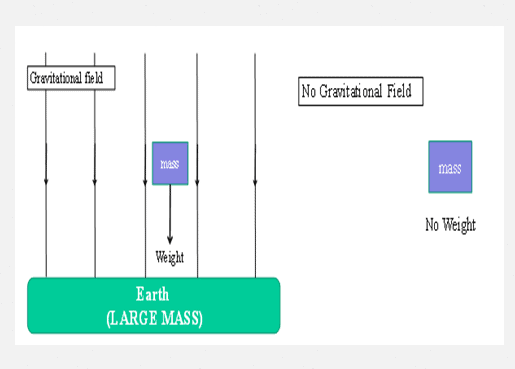Weight = force of gravity exerted on an object (or the force on a supporting scale)

Weight (N) = mass (kg) x g (N/kg)  g=gravitational field strength

b) describe qualitatively the motion of bodies falling in a uniform gravitational field with air resistance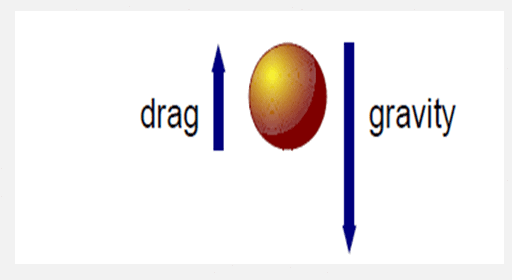When any object moves through air, the air offers a frictional resistance (drag) to the motion. This causes the object to decelerate. The deceleration is not constant but depends on the velocity of the object. The faster the object the greater the resistance and deceleration. You can experience this when you run – the faster you run, the harder the air seems to blow against.

Therefore If a body falls under gravity, air resistance opposes the fall and the downward acceleration is therefore reduced. This means that bodies falling through air take longer to fall the same distance than in vacuum

state the principle of conservation of momentum

When objects of a system interact, their total momentum before and after interaction are equal if no net (external) force acts on the system

The total momentum of an isolated system is constant

m1 u1 + m2 u2 = m1 v1 + m2 v2 if net F = 0 {for all collisions }

NB: Total momentum DURING the interaction/collision is also conserved

(Perfectly) elastic collision:

Both momentum & kinetic energy of the system are conserved.

Inelastic collision:

Only momentum is conserved, total kinetic energy is not conserved and the particles stick together after collision (i.e. move with the same velocity)In inelastic collisions, total energy is conserved but Kinetic Energy may be converted into other forms of energy such as sound and heat energy

Recommended: Solved questions on dynamics

Question 1

A ball X and a ball Y are travelling along the same straight line in the same direction, as
shown in the fig below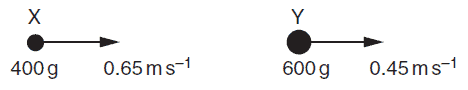Ball X has mass 400 g and horizontal velocity 0.65 m s–1.
Ball Y has mass 600 g and horizontal velocity 0.45 m s–1.
Ball X catches up and collides with ball Y. After the collision, X has horizontal velocity 0.41 m s–1
and Y has horizontal velocity v, as shown below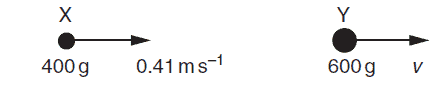Calculate
(i) the total initial momentum of the two balls,
(ii) the velocity v,
(iii) the total initial kinetic energy of the two balls.
(iv) Explain how you would check whether the collision is elastic

(v) Use Newton’s third law to explain why, during the collision, the change in momentum of X is
equal and opposite to the change in momentum of Y.

Before collision, the balls are moving in the same direction, therfore,

total momentum before collision = m1u1+ m2u2

= 0.4*0.65 + 0.6*0.45 = 0.53kgms-1

the total initial momentum of the two balls = 0.53kgms-1

After collision, the balls also moved in the same direction

total momentum after collision = m1v1+m2v2

= 0.4*0.41 + 0.6*v

total momentum before collision = total momentum after collision (law of conservation of linear momentum)

0.53 = 0.16 +0.6v

0.6v = 0.37

v = 0.37/0.6

v = 0.617

iii) the total initial kinetic energy of the two balls

1/2 m1 u1^2 +1/2 m2u2^2

1/2 *0.4*0.65^2 +1/2 *0.6*o.45^2

0.145 J

iv) how you would check whether the collision is elastic

check whether the relative speed of approach equals relative speed of separation

or

total final kinetic energy equals the total initial kinetic energy

v) Use Newton’s third law to explain why, during the collision, the change in momentum of X is
equal and opposite to the change in momentum of Y

Newton’s Third Law
When object X exerts a force on object Y, object Y exerts a force of the same type that is equal in magnitude and opposite in direction on object X.

Change in momentum of x = m1(v-u) = 0.4(0.41-0.65) = -0.1kgms-1

F = dp/dt

Change in momentum of y = m2(v-u) = 0.6(0.617-0.45) = 0.1kgms-1

the two balls will have the same time of impact during collision

therefore -Fx = Fy ………. this satisfy Newton’s third law of motion

It can be put in this way:

the forces on the two bodies (or on X and Y) are equal and opposite
time same for both forces and force is change in momentum / time

Two balls X and Y are supported by long strings, as shown below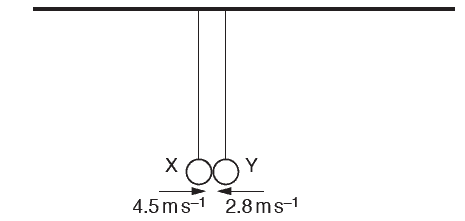The balls are each pulled back and pushed towards each other. When the balls collide at the
position shown in Fig. 3.1, the strings are vertical. The balls rebound in opposite directions

Figure below shows data for X and Y during this collision.The positive direction is horizontal and to the right.
Use the conservation of linear momentum to determine the mass M of Y.

From the key points given

m1u1 +m2u2  = m1v1 +m2v2

In the question given, before coollision the objects move in opposite direction toward each other and that is the reason for the negative velocity included in the question

therefore, total mometum before collision is

m1u1 – m2u2 = 0.05 *4.5 – M *2.8

note that the mass is in gramm(50g) but it has been converted to kilogramm (0.05), so that all the unit will be in S.I base unit.

After collision, the total momentum is

m2v2 -m2v2 = M* 1.4 – 0.05 *1.8

from law of conservation of linear momentum

0.05 *4.5 – M *2.8 = M* 1.4 – 0.05 *1.8

collecting like terms

0.225 +0.09 = 4.2M

0.315 = 4.2M

M = 0.315/4.2

M = 0.075Kg 0r 75g

A child on a sledge slides down a hill with acceleration a. The hill makes an angle θ with the
horizontal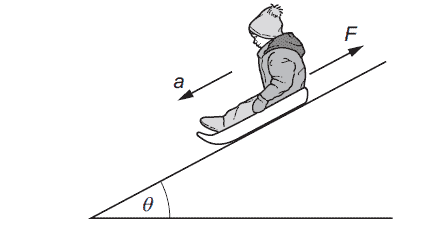The total mass of the child and the sledge is m. The acceleration of free fall is g.
What is the friction force F ?
A m(g cosθ – a)
B m(g cosθ + a)
C m(g sinθ – a)
D m(g sinθ + a)

According to Newton’s first law of motion

Object remain at rest or in a straight line motion with constant velocity, unless acted upon by external unbalanced forces

so, therefore we have two unbalanced forces acting on the boy, which are force down the slope and the frictional force.

The body accelerate uniformly down the slope because of there is a resultant force or net force

the net force = ma

therefore,

F -Fr = ma

F is the force down the slope

Fr is the frictional force opposing the motion of the boy

F = mgsinθ

mgsinθ – Fr = ma

Fr  = mgsinθ -ma

factorise, we then have

Fr = m(gsinθ -a)